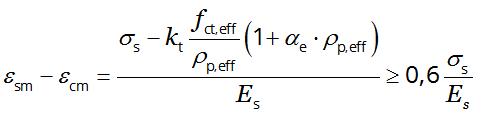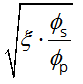For full functionality of this site it is necessary to enable JavaScript. Here are the instructions how to enable JavaScript in your web browser.

# Calculation of crack widths wk

## Eurocode 2 part 1-1: Design of concrete structures \$(document).ready(function () { var freeExample = \$("#freeExample").length; \$("#mainMenu li#nav-appCate, #mainMenu li#nav-appEC211").addClass("active"); var proUser = false === true || false === true || freeExample !== 0; if (!proUser) { \$('#Input input').keyup(function(){ \$('#AlertSubscribe').modal(); }); \$('#Input select').change(function(){ \$('#AlertSubscribe').modal(); }); }; var tempInput = \$("#tempInput").text(); if (tempInput != "") { \$("#Input").parent("form").deserialize(tempInput); if (proUser) { \$("#acceptBtn").trigger("click"); } }; });  7.3 Crack control

The crack width, wk, may be calculated as follows:

 wk = sr,max⋅(εsm - εcm) (7.8)

where:

sr,max
is the maximum crack spacing
εsm
is the mean strain in the reinforcement under the relevant combination of loads, including the effect of imposed deformations and taking into account the effects of tension stiffening
εcm
is the mean strain in the concrete between cracks.(7.9)

where:

σs
is the stress in the tension reinforcement assuming a cracked section,
see application for a rectangular section or application for a T-section
Es
is the design value of the modulus of elasticity of the reinforcing steel, see § 3.2.7 (4)
αe
is the ratio Es/Ecm

with

Ecm
the secant modulus of elasticity of concrete
fct,eff
is the mean value of the tensile strength of the concrete effective at the time when the cracks may first be expected to occur:
fct,eff = fctm or lower, (fctm(t)), if cracking is expected earlier than 28 days
ρp,eff
 = (As + ξ1⋅A'p)/Ac,eff (7.10)

with

As
the cross sectional area of reinforcement
A'p
the area of pre or post-tensioned tendons within Ac,eff
Ac,eff
the effective area of concrete in tension surrounding the reinforcement or prestressing tendons of depth, hc,ef, where hc,ef is the lesser of 2,5(h - d), (h - x)/3 or h/2 (see Figure 7.1)
ξ1
the adjusted ratio of bond strength taking into account the different diameters of prestressing and reinforcing steel:
 ξ1 =(7.5)

with

ξ
the ratio of bond strength of prestressing and reinforcing steel, according to Table 6.2
ΦS
the largest bar diameter of the reinforcing steel
ΦP
the diameter or equivalent diameter of prestressing steel:
Φp = 1,6⋅√AP for bundles, where AP is the area of a prestressing steel,
Φp = 1,75⋅Φwire for single 7 wire strands,
Φp = 1,20⋅Φwire for single 3 wire strands, where Φwire is the wire diameter.
kt
is a factor dependent on the duration of the load:

• Where the bonded reinforcenlent is fixed at reasonably close centres within the tension zone (spacing ≤ 5(c + Φ/2), cf. Figure 7.2), the maximum crack spacing sr,max may be calculated as follows:

 sr,max = k3c + k1k2k4Φ / ρp,eff (7.11)

where:

Φ
is the bar diameter. Where a mixture of bar diameters is used in a section, an equivalent diameter, Φeq, should be used.
c
is the cover to the longitudinal reinforcement
ρp,eff
see the difference of the mean strains above
k1
is a coefficient which takes account of the bond properties of the bonded reinforcement:
k1 = 0,8 for high bond bars,
k1 = 1,6 for bars with an effectively plain surface (e.g. prestressing tendons).
k2
is a coefficient which takes account of the distribution of strain:
k2 = 0,5 for bending,
k2 = 1,0 for pure tension.
Intermediate values of k2 should be used for cases of eccentric tension or for local areas:
 k2 = (ε1 + ε2)/(2ε1) (7.13)

where ε1 is the greater and ε2 is the lesser tensile strain at the boundaries of the section considered, assessed on the basis of a cracked section.

k3
is a Nationally Determined Parameter, see § 7.3.4 (3)
k4
is a Nationally Determined Parameter, see § 7.3.4 (3).

• Where the spacing of the bonded reinforcement exceeds 5(c + Φ/2) (cf. Figure 7.2), or where there is no bonded reinforcement within the tension zone, the maximum crack spacing sr,max may be calculated as follows:

 sr,max = 1,3(h - x) (7.14)

where:

h
is the overall depth of the section (see Figure 7.1)
x
is the neutral axis depth of the section (see Figure 7.1).

This application calculates the crack width wk from your inputs. Intermediate results will also be given.

First, change the following option if necessary:

Input
MPa
mm2
mm
mm
MPa
mm2
mm
MPa
mm
mm2
MPa

Output
αe
ξ1
(7.5)
ρp,eff
(7.10)
εsm - εcm
(7.9)
sr,max
mm (7.11)
the crack width wk
mm (7.8)